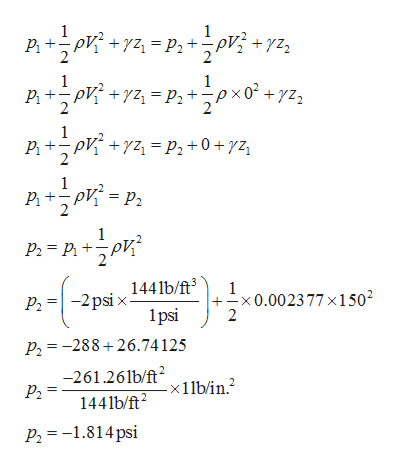# At a given point on a horizontal streamline in flowing air, the static ressureis -2.0 psi (i.e. a vacuum) and the velocity is 150 ft/s. Determine the pressure at a stagnation point on that streamline. [Answer in units of psi]

Question
At a given point on a horizontal streamline in flowing air, the static ressure
is -2.0 psi (i.e. a vacuum) and the velocity is 150 ft/s. Determine the pressure at a stagnation point on that streamline. [Answer in units of psi]
check_circleExpert Solution
Step 1

Given:

The static pressure p1 at the given point is -2.0 psi.

The velocity V1 at the given point is 150 ft/sec.

Step 2

Assume:

The density of the air ρ is 0.002377 sugs/ft3.

The velocity V2 at the stagnation point is zero.

The pressure at the stagnation point is p2.

The datum head of the given point and the stagnation point is z1 and z2.

As the streamline is horizontal, z1 and z2 are equal.

Step 3

Apply Bernoulli’s Equation at a given p...help_outlineImage TranscriptioncloseP +2P? + yz 1 1 1 Pp p2+0+yz_ 1 1441b/ft 1 -x 0.0023 77 x1502 2 2 psi x psi Р. P2=-288+26.74 125 -261.26lb/ft Р. 1441b/ft -x 1 lb/in.2 P2=-1.814psi fullscreen

### Want to see the full answer?

See Solution

#### Want to see this answer and more?

Solutions are written by subject experts who are available 24/7. Questions are typically answered within 1 hour*

See Solution
*Response times may vary by subject and question
Tagged in

### Civil Engineering